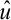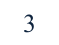Study Guides (400,000)
US (230,000)
KSU (400)
BUS (10)
Final

# BUS 10123 Chapter Notes - Chapter 9: Autoregressive Conditional Heteroskedasticity, Autoregressive Integrated Moving Average, Conditional VarianceExam

Department
Course Code
BUS 10123
Professor
Eric Von Hendrix
Study Guide
Final

This preview shows pages 1-3. to view the full 11 pages of the document.1. (a). A number of stylised features of financial data have been suggested at
the start of Chapter 9 and in other places throughout the book:
-Frequency: Stock market prices are measured every time there is a trade or
somebody posts a new quote, so often the frequency of the data is very high
-Non-stationarity: Financial data (asset prices) are covariance non-stationary;
but if we assume that we are talking about returns from here on, then we can
validly consider them to be stationary.
-Linear Independence: They typically have little evidence of linear
(autoregressive) dependence, especially at low frequency.
-Non-normality: They are not normally distributed they are fat-tailed.
-Volatility pooling and asymmetries in volatility: The returns exhibit volatility
clustering and leverage effects.
Of these, we can allow for the non-stationarity within the linear (ARIMA)
framework, and we can use whatever frequency of data we like to form the
models, but we cannot hope to capture the other features using a linear
model with Gaussian disturbances.
(b) GARCH models are designed to capture the volatility clustering effects in
the returns (GARCH(1,1) can model the dependence in the squared returns,
or squared residuals), and they can also capture some of the unconditional
leptokurtosis, so that even if the residuals of a linear model of the form given
by the first part of the equation in part (e), the
t
u
ˆ
’s, are leptokurtic, the
standardised residuals from the GARCH estimation are likely to be less
leptokurtic. Standard GARCH models cannot, however, account for leverage
effects.
(c) This is essentially a “which disadvantages of ARCH are overcome by
GARCH” question. The disadvantages of ARCH(q) are:
- How do we decide on q?
- The required value of q might be very large
- Non-negativity constraints might be violated.
When we estimate an ARCH model, we require
i >0 i=1,2,...,q (since variance cannot be negative)
GARCH(1,1) goes some way to get around these. The GARCH(1,1) model has
only three parameters in the conditional variance equation, compared to q+1
for the ARCH(q) model, so it is more parsimonious. Since there are less
parameters than a typical qth order ARCH model, it is less likely that the
estimated values of one or more of these 3 parameters would be negative

Only pages 1-3 are available for preview. Some parts have been intentionally blurred.2
than all q+1 parameters. Also, the GARCH(1,1) model can usually still capture
all of the significant dependence in the squared returns since it is possible to
write the GARCH(1,1) model as an ARCH(), so lags of the squared residuals
back into the infinite past help to explain the current value of the conditional
variance, ht.
(d) There are a number that you could choose from, and the relevant ones
that were discussed in Chapter 9, including EGARCH, GJR or GARCH-M.
The first two of these are designed to capture leverage effects. These are
asymmetries in the response of volatility to positive or negative returns. The
standard GARCH model cannot capture these, since we are squaring the
lagged error term, and we are therefore losing its sign.
The conditional variance equations for the EGARCH and GJR models are
respectively:
+++=
2
)log()log(
1
1
1
1
2
1
2
t
t
t
t
tt
u
u
and
t2 =
0 +
1
ut1
2
+

t-12+
ut-12It-1
where It-1 = 1 if ut-1 0
= 0 otherwise
For a leverage effect, we would see
> 0 in both models.
The EGARCH model also has the added benefit that the model is expressed in
terms of the log of ht, so that even if the parameters are negative, the
conditional variance will always be positive. We do not therefore have to
artificially impose non-negativity constraints.
One form of the GARCH-M model can be written
yt =
+other terms +

t-1+ ut , ut N(0,ht)
t2 =
0 +
1
ut1
2
+

t-12
so that the model allows the lagged value of the conditional variance to
affect the return. In other words, our best current estimate of the total risk of
the asset influences the return, so that we expect a positive coefficient for
.
Note that some authors use

t (i.e. a contemporaneous term).
(e). Since yt are returns, we would expect their mean value (which will be
given by
) to be positive and small. We are not told the frequency of the
data, but suppose that we had a year of daily returns data, then
would be

Only pages 1-3 are available for preview. Some parts have been intentionally blurred.3
the average daily percentage return over the year, which might be, say 0.05
(percent). We would expect the value of
0 again to be small, say 0.0001, or
something of that order. The unconditional variance of the disturbances
would be given by
0/(1-(
1 +
2)). Typical values for
1 and
2 are 0.8 and
0.15 respectively. The important thing is that all three alphas must be
positive, and the sum of
1 and
2 would be expected to be less than, but
close to, unity, with
2 >
1.
(f) Since the model was estimated using maximum likelihood, it does not
seem natural to test this restriction using the F-test via comparisons of
residual sums of squares (and a t-test cannot be used since it is a test
involving more than one coefficient). Thus we should use one of the
approaches to hypothesis testing based on the principles of maximum
likelihood (Wald, Lagrange Multiplier, Likelihood Ratio). The easiest one to
use would be the likelihood ratio test, which would be computed as follows:
1. Estimate the unrestricted model and obtain the maximised value of the
log-likelihood function.
2. Impose the restriction by rearranging the model, and estimate the
restricted model, again obtaining the value of the likelihood at the new
optimum. Note that this value of the LLF will be likely to be lower than the
unconstrained maximum.
3. Then form the likelihood ratio test statistic given by
LR = -2(Lr - Lu) 2(m)
where Lr and Lu are the values of the LLF for the restricted and
unrestricted models respectively, and m denotes the number of
restrictions, which in this case is one.
4. If the value of the test statistic is greater than the critical value, reject the
null hypothesis that the restrictions are valid.
(g) In fact, it is possible to produce volatility (conditional variance) forecasts
in exactly the same way as forecasts are generated from an ARMA model by
iterating through the equations with the conditional expectations operator.
We know all information including that available up to time T. The answer to
this question will use the convention from the GARCH modelling literature to
denote the conditional variance by ht rather than
t2. What we want to
generate are forecasts of hT+1 T, hT+2 T, ..., hT+s T where T denotes
all information available up to and including observation T. Adding 1 then 2
then 3 to each of the time subscripts, we have the conditional variance
equations for times T+1, T+2, and T+3:
hT+1 =
0 +
1
2
T
u
+
hT (1)